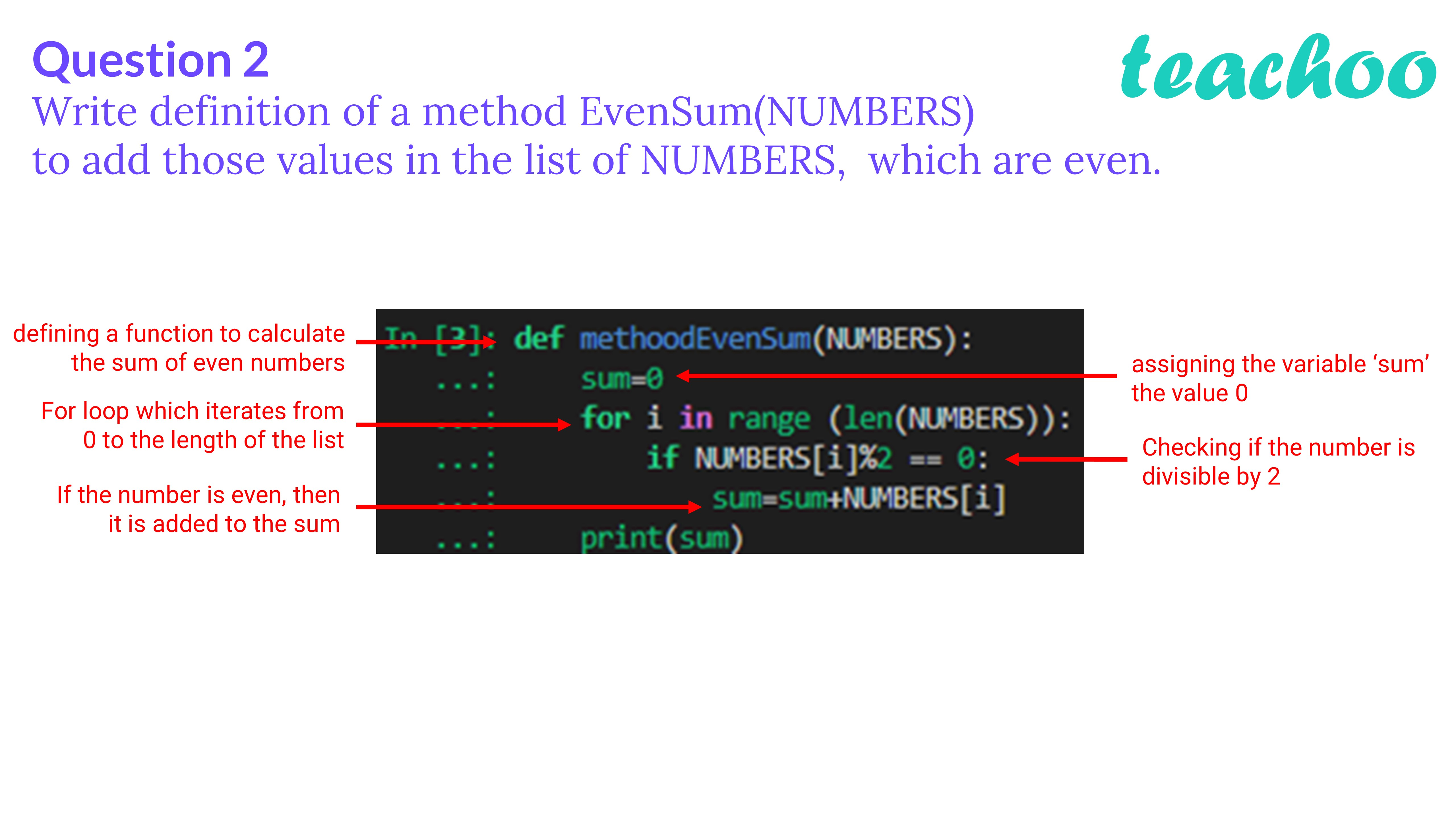Past Year - 3 Mark Questions

Computer Science - Class 12
Chapter 14 - Functions in python

## Write definition of a method Even Sum(NUMBERS) to add those values in the list of NUMBERS, which are even.

``` def methoodEvenSum (NUMBERS): ```

```     sum= 0 ```

```     for i in range (len(NUMBERS)): ```

```         if NUMBERS[i]% 2 == 0 : ```

```             sum=sum+NUMBERS[i] ```

```     print(sum) ```Learn in your speed, with individual attention - Teachoo Maths 1-on-1 Class

### Transcript

Question 2 Write definition of a method EvenSum(NUMBERS) to add those values in the list of NUMBERS, which are even. defining a function to calculate the sum of even numbers For loop which iterates from 0 to the length of the list If the number is even, then it is added to the sum assigning the variable ‘sum’ the value 0 Checking if the number is divisible by 2